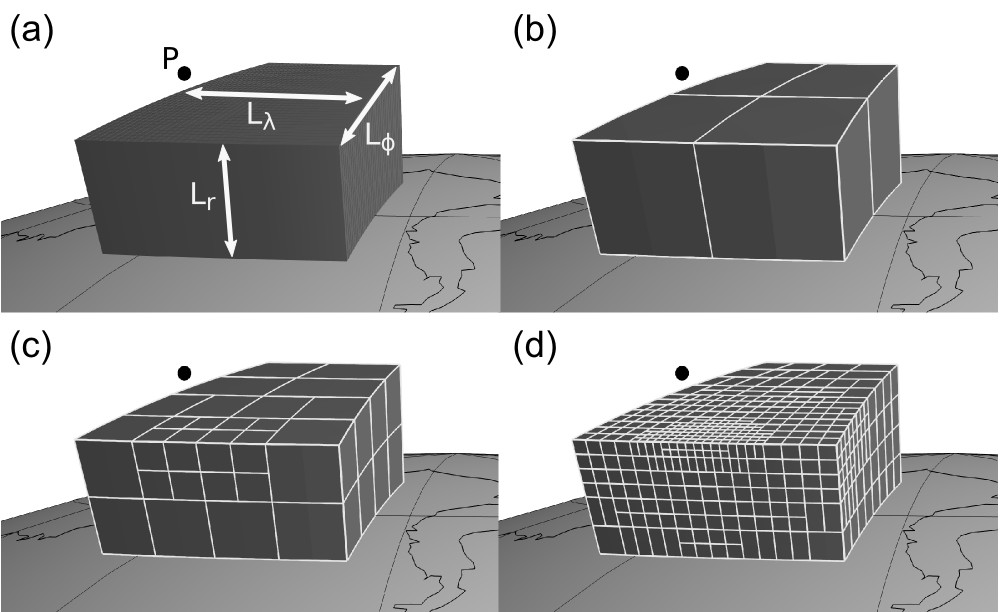# Tesseroids: forward modeling gravitational fields in spherical coordinates

Leonardo Uieda, Valéria C. F. Barbosa, Carla Braitenberg

This paper describes the algorithms used in version 1.2.0 of the open-source software Tesseroids. The software is a suite of C coded command-line programs that calculate the gravitational field of a tesseroid (spherical prism) model. There is also a separate Python implementation of the same algorithm in the `fatiando.gravmag.tesseroid` module of the Fatiando a Terra library (introduced in version 0.3) and in Harmonica.Figure: Adaptive discretization of the tesseroid shown in panel (a) for a computation point P using the distance-size ratio D equal to (b) 1, (c) 2, and (d) 6. Lr, Lφ, and Lλ are the dimensions of the tesseroid. Note that increasing D results in a fine division of the tesseroid close the computation point and a coarser division further away.

## Abstract

We present the open-source software Tesseroids, a set of command-line programs to perform the forward modeling of gravitational fields in spherical coordinates. The software is implemented in the C programming language and uses tesseroids (spherical prisms) for the discretization of the subsurface mass distribution. The gravitational fields of tesseroids are calculated numerically using the Gauss-Legendre Quadrature (GLQ). We have improved upon an adaptive discretization algorithm to guarantee the accuracy of the GLQ integration. Our implementation of adaptive discretization uses a "stack" based algorithm instead of recursion to achieve more control over execution errors and corner cases. The algorithm is controlled by a scalar value called the distance-size ratio (D) that determines the accuracy of the integration as well as the computation time. We determined optimal values of D for the gravitational potential, gravitational acceleration, and gravity gradient tensor by comparing the computed tesseroids effects with those of a homogeneous spherical shell. The values required for a maximum relative error of 0.1% of the shell effects are D = 1 for the gravitational potential, D = 1.5 for the gravitational acceleration, and D = 8 for the gravity gradients. Contrary to previous assumptions, our results show that the potential and its first and second derivatives require different values of D to achieve the same accuracy. These values were incorporated as defaults in the software.

## Citation

Uieda, L., V. Barbosa, and C. Braitenberg (2016), Tesseroids: Forward-modeling gravitational fields in spherical coordinates, GEOPHYSICS, F41–F48, doi:10.1190/geo2015-0204.1.